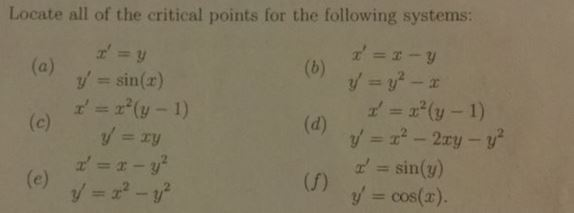Locate all of the critical points for the following systems:y' = sin(r)x' = x"(y-1)z' = x"(y-1)sin(y)(f)y' = cos(x)

Question

pls explain to me step by step and dont skip any steps. Thankshelp_outlineImage TranscriptioncloseLocate all of the critical points for the following systems: y' = sin(r) x' = x"(y-1) z' = x"(y-1) sin(y) (f) y' = cos(x) fullscreen
Step 1

Hey, since there are multiple sub-parts posted, we will answer first three sub-parts. If you want any specific sub-parts to be answered then please submit those sub-parts or specify the sub-part number in your message.

To  find the critical points for the given system of equations, equate y' = 0 and x' = 0. Find the corresponding (x,y) coordinates. It will be the critical points.

Step 2

(a) Find the critical points for the given system.

Step 3

(b) Find the critical points for th...

Want to see the full answer?

See Solution

Want to see this answer and more?

Our solutions are written by experts, many with advanced degrees, and available 24/7

See Solution
Tagged in

Math